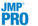Predictive and Specialized Modeling > Boosted Tree > Overview of the Boosted Tree Platform
Publication date: 07/30/2020

#Overview of the Boosted Tree Platform

The Boosted Tree platform produces an additive decision tree model that is based on many smaller decision trees that are constructed in layers. The tree in each layer consists of a small number of splits, typically five or fewer. Each layer is fit using the recursive fitting methodology described in Partition Models. The only difference is that fitting stops at a specified number of splits. For a given tree, the predicted value for an observation in a leaf is the mean of all observations in that leaf.

The fitting process proceeds as follows:

1. Fit an initial layer.

2. Compute residuals. These are obtained by subtracting the predicted mean for observations within a leaf from their actual value.

3. Fit a layer to the residuals.

4. Construct the additive tree. For a given observation, sum its predicted values over the layers.

5. Repeat step 2 to step 4 until the specified number of layers is reached, or, if validation is used, until fitting an additional layer no longer improves the validation statistic.

The final prediction is the sum of the predictions for an observation over all the layers.

By fitting successive layers on residuals from previous layers, each layer can improve the fit.

For categorical responses, only those with two response levels are supported. For a categorical response, the residuals fit at each layer are offsets of linear logits. The final prediction is a logistic transformation of the sum of the linear logits over all the layers.